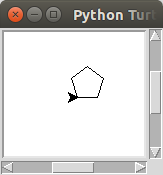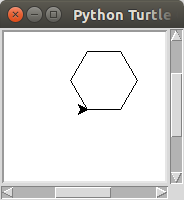# CPSC150AScientific Computing

## Activity 3

Loops

### Twenty Five to Fifty

Write a python program that uses a for loop to print all of the numbers between 25 and 50.

#### Example

25
26
27
... omitted for space ...
49
50

### Multiples of Seven

Write a python program that uses a for loop to print all multiples of 7 less than 100.

#### Example

7
14
21
... omitted for space ...
91
98

### Polygon

A convex regular polygon is a shape that consists of a sequence of straight lines that all have the same length and meet at the same angle. Convex regular polygons can be parameterized according to the number of sides the shape has. For example, the convex regular polygons with 3, 4, and 5 sides are an equilateral triangle, a square, and a pentagon. As the number of sides increases the names of the polygons get more complicated, for example a 36-sided convex regular polygon is called a triacontakaihexagon. In this activity you will create a function that can create any convex regular polygon.

#### Details

Create a Python program that uses turtle graphics to draw a convex regular polygon. It should prompt the user for the number of sides and for the circumference of the polygon it draws.

Test your program by running it multiple times with different input values.

#### Example

Enter the number of sides: 5
Enter the circumference: 100Enter the number of sides: 6 Enter the circumference: 200#### Hint

• Drawing a convex regular polygon is a lot like drawing a star, the turtle should repeatedly move forward and turn.
• Unlike the star, you will need to use a for loop to draw the polygon. Because the program must work for any number of sides, the number of times the turtle moves forward and turns will be different each time the program is run. Use the number of sides entered by the user, don’t forget to make it an int, to control the number of times the loop runs.
• Also just like drawing the star, the turtle will need to turn once for each corner of the polygon. So you can calculate how much it should turn because the sum of all of the turns will total 360°.
• Calculate the distance the turtle moves forward from the circumference of the polygon. The turtle will move forward the same distance every time, so the sum of every forward movement must be the circumference of the polygon.

### Spiral

The human visual system did not evolve to percieve the types of regular patterns that are simple to create with programming. A spiral of contrasting colors can produce the illusion of motion when you move your eyes over the static image.

#### Details

Create a Python program that uses turtle graphics to draw a square spiral. The spiral can be any size, but it looks cooler if it is bigger with little distance between each layer of the spiral.